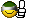Page 1 of 1

### Changing ammo count

Posted: May 18th '18, 00:21
I am having some issues with changing the way ammo shows up in the vanilla HUD for my scenario.

For some of my weapons, I want to put in graphics for something instead of the box like the Fusion Gun has. I tried to change this with MML but even when I tell it to pull graphics instead of a box, it still shows up in the game.

### Re: Changing ammo count

Posted: May 18th '18, 02:28
It might be easier to resolve the issue if you post the MML you're using. Could be a syntax error (I've definitely had those before - probably still have a few). It's sometimes difficult to tell about those in scripting languages where there's no compiler, per se.

### Re: Changing ammo count

Posted: May 20th '18, 20:29
The Man wrote:It might be easier to resolve the issue if you post the MML you're using. Could be a syntax error (I've definitely had those before - probably still have a few). It's sometimes difficult to tell about those in scripting languages where there's no compiler, per se.
<interface>

<!-- TR -->
<weapon index="2" shape="39" left="440" top="370">
<ammo index="0" type="2" left="390" top="370" across="100" delta_x="4" delta_y="10" bullet_shape="57" empty_shape"58" right_to_left="1" />
<ammo index="1" type="2" left="390" top="370" across="100" delta_x="4" delta_y="10" bullet_shape="57" empty_shape"58" right_to_left="1" />
</weapon>

</interface>

### Re: Changing ammo count

Posted: May 21st '18, 04:00
I’m in a rather precarious state of mind right now for reasons I won’t bore you with, so I’m not certain I understand exactly what you want that to do or exactly why it isn’t working, but I find that, when in doubt, it usually pays to look at other people’s working code. Phoenix did something almost identical with some of its weaponry; here’s its IMP55 code.

Code: Select all

``````  <!-- Pistol to IMP55 -->
<weapon index="1" shape="37" left="390" multiple="1" multiple_shape="38" multiple_unusable_shape="37" multiple_delta_x="0" multiple_delta_y="0">
<ammo index="0" bullet_shape="45" empty_shape="46" left="490" top="390" across="32" down="1" delta_x="3" delta_y="7" type="2" />
<ammo index="1" bullet_shape="45" empty_shape="46" left="490" top="375" across="32" down="1" delta_x="3" delta_y="7" type="2" />
</weapon>
``````
One thing that immediately jumps out at me in your code is that it’s missing equal signs between each instance of empty_shape and the subsequent inverted commas. I’m not sure if that’s taken from your script or if they somehow got deleted when you copied them over, but if they’re not in your script, that’s your problem (or at least one of them). I haven’t actually tested this because without your shapes file it probably won’t work as intended either way, but it should be:

Code: Select all

`````` <interface>

<!-- TR -->
<weapon index="2" shape="39" left="440" top="370">
<ammo index="0" type="2" left="390" top="370" across="100" delta_x="4" delta_y="10" bullet_shape="57" empty_shape="58" right_to_left="1" />
<ammo index="1" type="2" left="390" top="370" across="100" delta_x="4" delta_y="10" bullet_shape="57" empty_shape="58" right_to_left="1" />
</weapon>

</interface>``````
(btw, [code]input[/code] will preserve the spacing of your code)

### Re: Changing ammo count

Posted: May 23rd '18, 00:31
Ugh it's always some stupid thing like that haha.

All is good now. Thanks for pointing that out!### Re: Changing ammo count

Posted: May 23rd '18, 02:30
No problem. Anyone who's written code has undoubtedly made syntax errors like that often. It helps to have a second pair of eyes.

Your scenario screenshots look cool. I'll probably say more at some point in the proper thread - limits on post speed prevent me from posting in both places before my break ends, and I'm on my phone, which limits how quickly I can type.